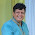## Wednesday, September 26, 2018

### Geometry Problem 1389: Triangle, 40-120-20 degree, Angle, Congruence

Geometry Problem. Post your solution in the comment box below.
Level: Mathematics Education, High School, Honors Geometry, College.

Details: Click on the figure below.1.Find E on AC such that AE = BE. Construct equilateral Tr. BEF, F within Tr. BCE

Since BC = AD = CE, AE = BE = EF = CD

So Tr.s CEF & BCD are congruent SAS

Hence < FCE = < CBD = 10 and so
x = 30

Sumith Peiris
Moratuwa
Sri Lanka

1.Sumith Sir,

You are truly an expert at geometry problems. You always provide very elegant solutions.

2.Thank u for your kind words Dinesh. I thank God for whatever small talent I may have

2.Let point E between A and D such as AE=BE.So <DBC=10.

3.Luăm punctul E pe prelungirea lui BC astfel încât CE = CA; triunghiul AEC este isoscel (80-20-80).
Ducem BF paralel cu EA și atunci se arată ușor că EB=BF=FA=CD(triunghiul ABF isoscel 40-100-40).
Construim un triunghi isoscel CDP cu unghiul DPC = 20.
Deoarece CD = BF, implică triunghiul CBF = CDP și de aici CP = CB. De asemeni unghiul BCP = 60, ceea ce face triunghiul BPC echilateral(60-60-60) și PB =PC=PD. În triunghiul BDP isoscel BP = DP și
unghiul BPD =40. De aici unghiul PDB = 70, adică unghiul BDC = 150 și deci BDA =30.
Florin Popa , Comanesti, Romania.

4.Considering usual triangle notations,
Mark a point E on AC such that CE=a => AE=x ------------(1)
Observe that BEC is an 80-80-20 isosceles and ABE is a 40-40-100 isosceles with AE=BE=x -----------(2)
Now extend the internal angle bisector of m(ABC) to a point P such that BP=a and this forms an equilateral triangle BPC
From this construction it can be observed that C is the circumcenter of EBP
=> m(BEP) = (360-m(BCP))/2 = 360-60/2 = 150 degrees----------(3)
Another observation is that EB=DC=x, m(EBP)=m(DCB)=20 & BP=BC=a => EBP is congruent to DCB (SAS)
=>m(CDB)=m(BEP)=150
=>m(BDA)=x=30 Q.E.D

5.Extend (AB to E so that triangle BCE be equilateral, take F on AC so that EF=EC, we get <EFC=<FCE=80, and <BEF=40, thus AF=EF and F=D, hence <AFB=<BEC/2=30.

6.Hello !

Construct a triangle PCD outside the triangle BDC , DPC=20° , PD=PC=BC.
PCB=60° and PBC is equilateral. So, PB=PD and BPD=40°. We conclude that DBP=BDP=70°. As we know, CBP=60°, so DBC=10°.
Finally, x=DBC+DCB=10°+20°=30°.

7.More general form: A is twice of C.
The many solution for 10-20.
https://artofproblemsolving.com/community/c6h487183p2729760